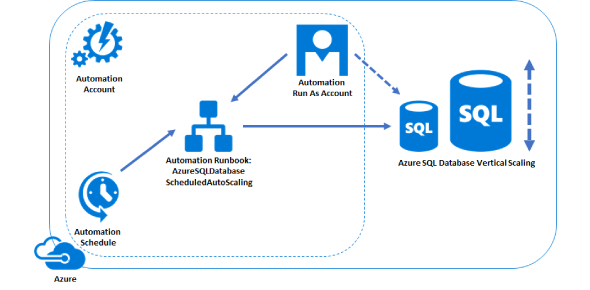# SQL Exam: Trivia Questions! Quiz

10 Questions | Total Attempts: 574SettingsSQL Exam: trivia questions quiz! The structured query language is designed to help a programmer manipulate databases. It helps in retrieval and editing of data from the database with ease. This computer language is one of the easiest to understand and was first created in the 1970s. By taking this quiz, you will get a chance to test out your knowledge of the language and how to write it. Give it a try!

Questions and Answers
• 1.
SQL < select name ,sal from Emp where name like m%;
• A.

True

• B.

False

• 2.
SQL < select name , job from Emp where sal < = 25000 or job = sales ;
• A.

True

• B.

False

• 3.
SQL < select name , job from Emp  where sal < = 25000 or job = 'sales' ;
• A.

True

• B.

False

• 4.
SQL < select * from Emp where sal between 1000 and 2000.
• A.

True

• B.

False

• 5.
SQL < select * from Emp where sal between 1000 and 2000 ;
• A.

True

• B.

False

• 6.
SQL < select  * where sal between 1000 and 2000 ;
• A.

True

• B.

False

• 7.
SQL < select * from Emp where sal between 1000 and 2000 ;
• A.

True

• B.

False

• 8.
SQL < select * from Emp where sal  1000 and 2000 ;
• A.

True

• B.

False

• 9.
SQL < select * from Emp where sal between 1000 and 2000 ;
• A.

True

• B.

False

• 10.
SQL < select name ,sal from Emp where name like 'm%';
• A.

True

• B.

False

Related Topics